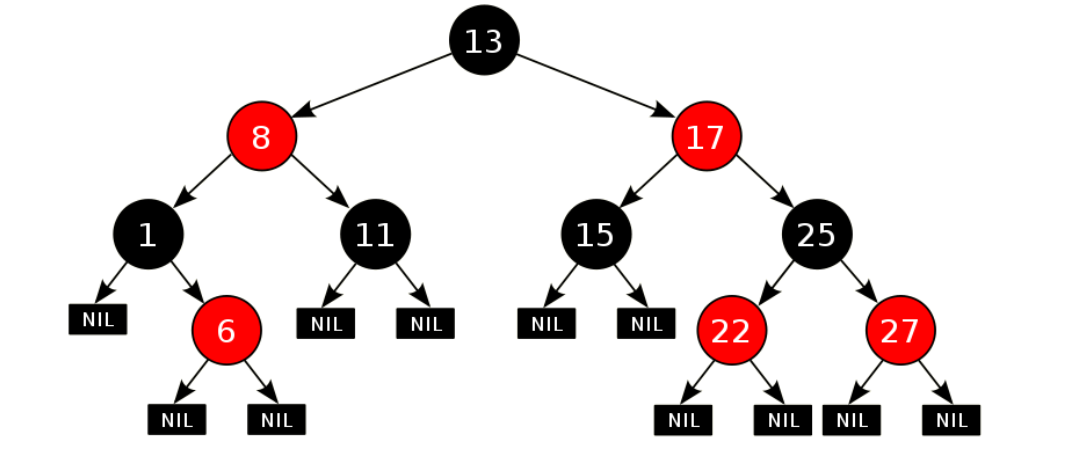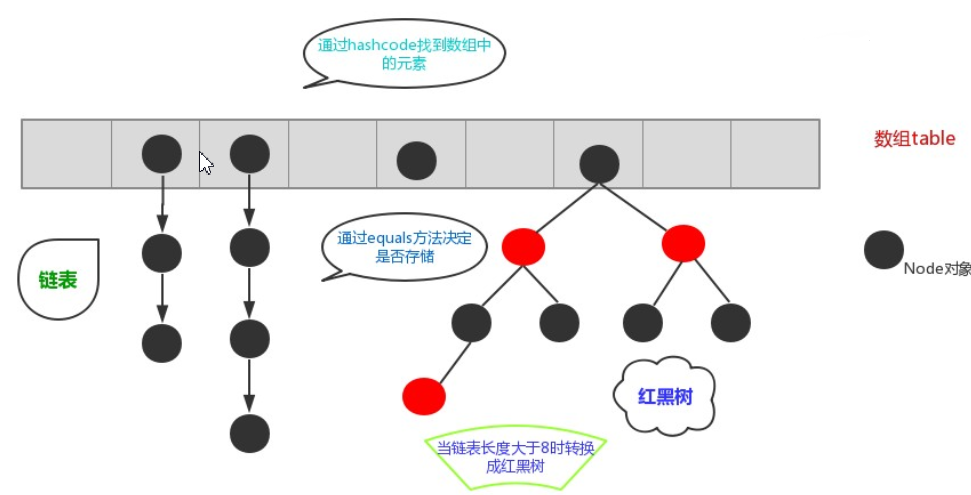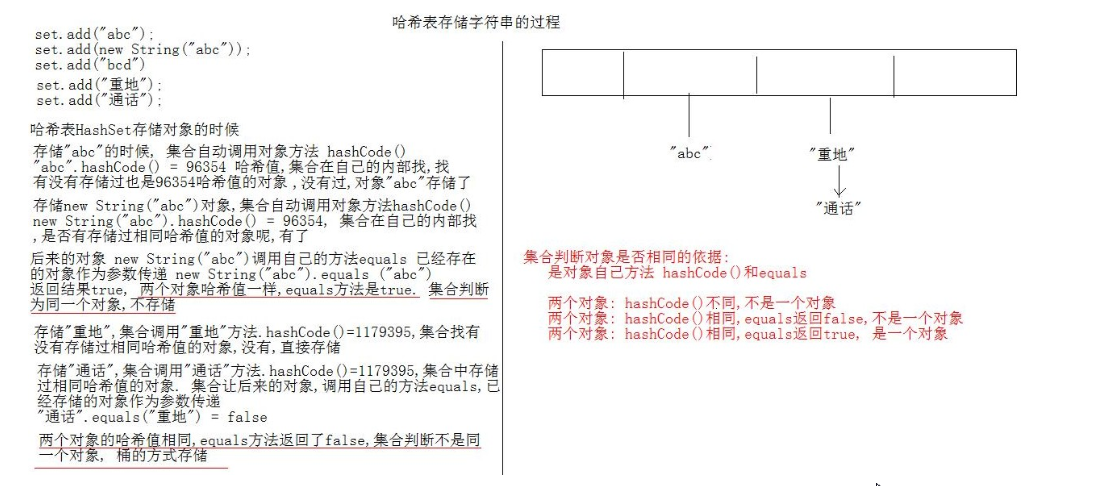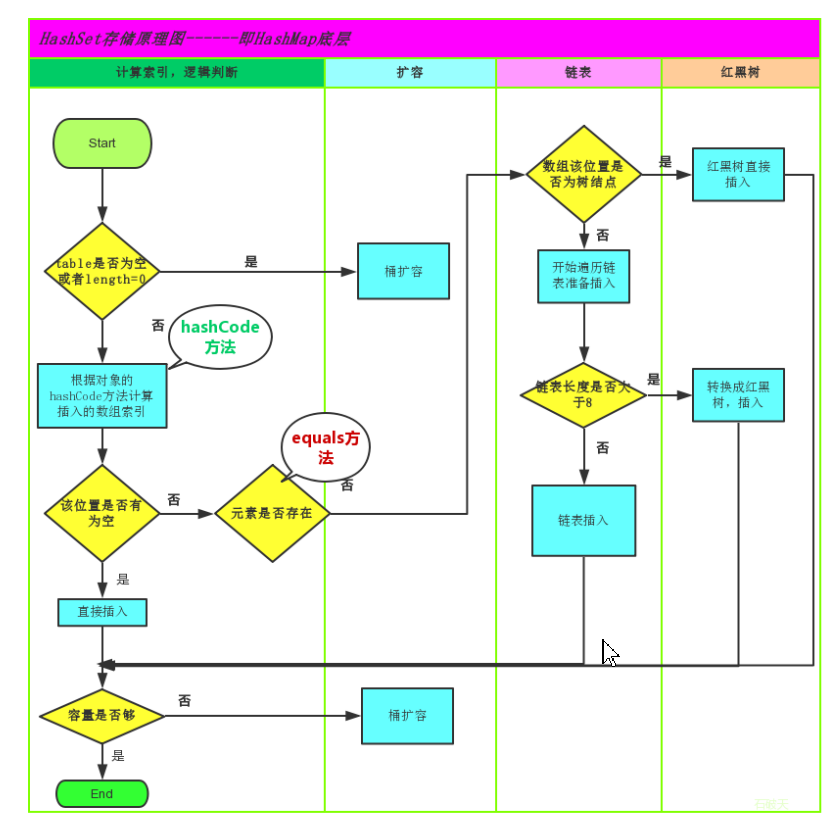# JavaSE第16篇：集合之Collection集合下篇

## 第一章：Collections工具类

### 1.1-Collections常用方法（记忆）

java.utils.Collections 是集合工具类，提供一系列静态方法用来对集合进行操作 。常用的方法如下：

1. public static <T> boolean addAll(Collection<T> c, T... elements) :往集合中添加一些元素。
2. public static void shuffle(List<?> list) 打乱顺序 :打乱集合顺序。
3. public static <T> void sort(List<T> list) :将集合中元素按照默认规则排序。
4. public static <T> void sort(List<T> list，Comparator<? super T> ):将集合中元素按照指定规则排序。

   ArrayList<Integer> list = new ArrayList<>();
//public static <T> boolean addAll(Collection<T> c, T... elements) :往集合中添加一些元素。
Collections.addAll(list, 1, 3, 4, 5, 6, 8, 7);
System.out.println(list); // [1, 3, 4, 5, 6, 8, 7]
//public static void shuffle(List<?> list) 打乱顺序 :打乱集合顺序。
Collections.shuffle(list);
System.out.println(list); // 随机顺序[5, 4, 6, 3, 7, 1, 8]
//public static <T> void sort(List<T> list) :将集合中元素按照默认规则排序。
Collections.sort(list);
System.out.println(list); // 默认升序[1, 3, 4, 5, 6, 7, 8]
//public static <T> void sort(List<T> list，Comparator<? super T> ) :将集合中元素按照指定规则排序。
Collections.sort(list, new Comparator<Integer>() {
@Override
public int compare(Integer o1, Integer o2) {
return o2-o1;
}
});
System.out.println(list);


### 1.2-比较器Comparator接口（理解）

public interface Comparator<T> 是一个比较功能，对一些对象的集合施加了一个整体排序。使用这个功能排序时，需要重写该接口中的方法：int compare(T o1, T o2)，其中o1和o2代表集合中两个要比较的元素，比较其两个参数的顺序。 返回负整数，零或正整数，因为第一个参数小于，等于或大于第二个参数。

Student学生类

public class Student{
private String name;
private int age;
//构造方法
//get/set
//toString
}


public static void main(String[] args) {
ArrayList<Student> list = new ArrayList<Student>();
Collections.sort(list, new Comparator<Student>() {
// 匿名内部类中重写Compare方法
@Override
public int compare(Student o1, Student o2) {
return o1.getAge()-o2.getAge();//以学生的年龄升序
// return o2.getAge() - o2.getAge();   // 倒序
}
});
}

for (Student student : list) {
System.out.println(student);
}
}
/*	 结果：
Student{name='jack', age=16}
Student{name='rose', age=18}
Student{name='abc', age=20}
*/


## 第二章：泛型

### 2.1-概述（了解）

package www.penglei666.com.Demo03;

import java.util.ArrayList;
import java.util.Collection;
import java.util.Iterator;

public class Test01 {
public static void main(String[] args) {
Collection coll = new ArrayList();
Iterator it = coll.iterator();
while(it.hasNext()){
//需要打印每个字符串的长度,就要把迭代出来的对象转成String类型
String str = (String) it.next();
System.out.println(str.length());
}
}
}



### 2.2-泛型的作用（了解）

• 将运行时期的ClassCastException，转移到了编译时期变成了编译失败。
• 避免了类型强转的麻烦。

public static void main(String[] args) {
Collection<String> list = new ArrayList<String>();
// 集合已经明确具体存放的元素类型，那么在使用迭代器的时候，迭代器也同样会知道具体遍历元素类型
Iterator<String> it = list.iterator();
while(it.hasNext()){
String str = it.next();
//当使用Iterator<String>控制元素类型后，就不需要强转了。获取到的元素直接就是String类型
System.out.println(str.length());
}
}


### 2.3-定义和使用（记忆）

修饰符 class 类名<代表泛型的变量> { }


class ArrayList<E>{

public E get(int index){ }
....
}


class ArrayList<String>{

public String get(int index){  }
...
}


class ArrayList<Integer> {
public boolean add(Integer e) { }

public Integer get(int index) {  }
...
}


修饰符 <代表泛型的变量> 返回值类型 方法名(参数){  }


public class MyGenericMethod {
public <MVP> void show(MVP mvp) {
System.out.println(mvp.getClass());
}

public <MVP> MVP show2(MVP mvp) {
return mvp;
}
}


public static void main(String[] args) {
// 创建对象
MyGenericMethod mm = new MyGenericMethod();
// 演示看方法提示
mm.show("aaa");
mm.show(123);
mm.show(12.45);
}


public interface MyGenericInterface<E>{

public abstract E getE();
}


public class MyImp1 implements MyGenericInterface<String> {
@Override
// 省略...
}

@Override
public String getE() {
return null;
}
}


public class MyImp2<E> implements MyGenericInterface<E> {
@Override
// 省略...
}

@Override
public E getE() {
return null;
}
}


public static void main(String[] args) {
MyImp2<String>  my = new MyImp2<String>();
}


### 2.4-泛型通配符（了解）

import java.util.ArrayList;
import java.util.Collection;

public class Test03 {
public static void main(String[] args) {
Collection<Integer> list1 = new ArrayList<>();
show(list1);
Collection<String> list2 = new ArrayList<>();
show(list2);
}
public static void  show(Collection<?> list) {
for (Object o : list) {
System.out.println(o);
}
}
}



• 格式类型名称 <? extends 类 > 对象名称
• 意义只能接收该类型及其子类

• 格式类型名称 <? super 类 > 对象名称
• 意义只能接收该类型及其父类型

package www.penglei666.com.Demo04;

/**
* 员工类
*/
public abstract class Employee {
private String name;
private int age;

public Employee() {
}

public Employee(String name, int age) {
this.name = name;
this.age = age;
}
public abstract void work();

public String getName() {
return name;
}

public void setName(String name) {
this.name = name;
}

public int getAge() {
return age;
}

public void setAge(int age) {
this.age = age;
}
}



package www.penglei666.com.Demo04;

public class Teacher extends Employee {
public Teacher(){}
public Teacher(String name,int age) {
super(name,age);
}
@Override
public void work() {
System.out.println(this.getName() + "老师，传道受业解惑");
}
}



package www.penglei666.com.Demo04;

public class Manger  extends Employee{
public Manger(){}
public Manger(String name,int age) {
super(name,age);
}
@Override
public void work() {
System.out.println(this.getName() + "老班，严格纪律管理班级");
}
}



package www.penglei666.com.Demo04;

import java.util.ArrayList;
import java.util.Collection;

public class Test {
public static void main(String[] args) {
Collection<Teacher> list1 = new ArrayList<>();
Collection<Manger> list2 = new ArrayList<>();
show(list1);
show(list2);
}
public static void show(Collection<? extends Employee> list){
for (Employee employee : list) {
employee.work();
}
}
}



## 第三章：数据结构-红黑树

### 3.1-什么是红黑树（了解）### 3.2-红黑树的约束和作用（了解）

1. 节点可以是红色的或者黑色的。
2. 根节点是黑色的。
3. 叶子节点(特指空节点)是黑色的。
4. 每个红色节点的子节点都是黑色的。
5. 任何一个节点到其每一个叶子节点的所有路径上黑色节点数相同。

## 第四章：Set集合

### 4.1-概述（了解）

java.util.Set接口和java.util.List接口一样，同样继承自Collection接口，它与Collection接口中的方法基本一致，并没有对Collection接口进行功能上的扩充，只是比Collection接口更加严格了。与List接口不同的是，Set接口下的集合不存储重复的元素。

Set集合有多个子类，这里我们介绍其中的java.util.HashSetjava.util.LinkedHashSet这两个集合。

### 4.2-Set集合的使用（重点）

public static void main(String[] args)  {
Set<String> set = new HashSet<String>();
Iterator<String> it = set.iterator();
while (it.hasNext()){
String str = it.next();
System.out.println(str);
}
for(String str : set){
System.out.println(str);
}
}


### 4.3-对象的哈希值（了解）

java.lang.Object类中定义了方法：public int hashCode()返回对象的哈希码值，任何类都继承Object，也都会拥有此方法。

public class Person{}


public static void main(String[] args){
Person person = new Person();
int code = person.hashCode();
}


142666848


Person类重写hashCode()方法：直接返回0

public int hashCode(){
return 0;
}


### 4.4-String对象的哈希值（了解）

public static void main(String[] args){
String s1 = new String("abc");
String s2 = new String("abc");
System.out.println(s1.hashCode());
System.out.println(s2.hashCode());
}


String类的hashCode方法源码分析

private final char value[];


/*
*  String类重写方法hashCode()
*  返回自定义的哈希值
*/
public int hashCode() {
int h = hash;
if (h == 0 && value.length > 0) {
char val[] = value;

for (int i = 0; i < value.length; i++) {
h = 31 * h + val[i];
}
hash = h;
}
return h;
}


hashCode源码分析：

• int h = hash，hash是成员变量，默认值0，int h = 0。
• 判断h==0为true && value.length>0，数组value的长度为3，保存三个字符abc，判断结果整体为true。
• char val[] = value，将value数组赋值给val数组。
• for循环3次，将value数组进行遍历，取出abc三个字符。
• 第一次循环： h = 31 * h + val，h = 31 * 0 + 97结果：h = 97。
• 第二次循环： h = 31 * 97 + val，h = 31 * 97 + 98结果：h = 3105。
• 第三次循环： h = 31 * 3105 + val，h = 31 * 3105 + 99结果：h = 96354。
• return 96354。
• 算法：31 * 上一次的哈希值+字符的ASCII码值，31属于质数，每次乘以31是为了降低字符串不同，但是计算出相同哈希值的概率。

### 4.5-哈希表（了解）

JDK1.8之前，哈希表底层采用数组+链表实现，即使用数组处理冲突，同一hash值的链表都存储在一个数组里。但是当位于一个桶中的元素较多，即hash值相等的元素较多时，通过key值依次查找的效率较低。而JDK1.8中，哈希表存储采用数组+链表+红黑树实现，当链表长度超过阈值（8）时，将链表转换为红黑树，这样大大减少了查找时间。

• 哈希表的初始化容量，数组长度为16个。
• 当数组容量不够时，扩容为原数组长度的2倍
• 加载因子为0.75。
• 指示当数组的容量被使用到长度的75%时，进行扩容。HashSet存储原理图### 4.6-HashSet集合的特点（了解）

• HashSet底层数据结构是单向哈希表
• 不保证元素的迭代顺序，存储元素的顺序和取出元素的顺序不一致。
• 此集合不允许存储重复元素。
• 存储在此集合中的元素应该重写hashCode()和equals()方法保证唯一性。
• 此集合具有数组，链表，红黑树三种结构特点。
• 线程不安全，运行速度快。

### 4.7-HashSet存储自定义类型（重点）

public class Student {
private String name;
private int age;

//get/set
@Override
public boolean equals(Object o) {
if (this == o)
return true;
if (o == null || getClass() != o.getClass())
return false;
Student student = (Student) o;
return age == student.age &&
Objects.equals(name, student.name);
}

@Override
public int hashCode() {
return Objects.hash(name, age);
}
}


public static void main(String[] args) {
//创建集合对象   该集合中存储 Student类型对象
HashSet<Student> stuSet = new HashSet<Student>();
//存储

for (Student stu2 : stuSet) {
System.out.println(stu2);
}
}


### 4.8-哈希表源代码分析（了解）

HashSet集合本身并不提供功能，底层依赖HashMap集合，HashSet构造方法中创建了HashMap对象：

map = new HashMap<>();


HashMap无参数构造方法的分析

//HashMap中的静态成员变量
static final float DEFAULT_LOAD_FACTOR = 0.75f;
public HashMap() {
}


HashMap有参数构造方法分析

public HashMap(int initialCapacity, float loadFactor) {
if (initialCapacity < 0)
throw new IllegalArgumentException("Illegal initial capacity: " +
initialCapacity);
if (initialCapacity > MAXIMUM_CAPACITY)
initialCapacity = MAXIMUM_CAPACITY;
throw new IllegalArgumentException("Illegal load factor: " +
this.threshold = tableSizeFor(initialCapacity);
}


• 如果initialCapacity（初始化容量）小于0，直接抛出异常。
• 如果initialCapacity大于最大容器，initialCapacity直接等于最大容器
• MAXIMUM_CAPACITY = 1 << 30 是最大容量 (1073741824)
• tableSizeFor（initialCapacity）方法计算哈希表的初始化容量。
• 注意：哈希表是进行计算得出的容量，而初始化容量不直接等于我们传递的参数。

tableSizeFor方法分析

static final int tableSizeFor(int cap) {
int n = cap - 1;
n |= n >>> 1;
n |= n >>> 2;
n |= n >>> 4;
n |= n >>> 8;
n |= n >>> 16;
return (n < 0) ? 1 : (n >= MAXIMUM_CAPACITY) ? MAXIMUM_CAPACITY : n + 1;
}


• 例如传递2，结果还是2，传递的是4，结果还是4。
• 例如传递3，结果是4，传递5，结果是8，传递20，结果是32。

Node 内部类分析

 static class Node<K,V> implements Map.Entry<K,V> {
final int hash;
final K key;
V value;
Node<K,V> next;
Node(int hash, K key, V value, Node<K,V> next) {
this.hash = hash;
this.key = key;
this.value = value;
this.next = next;
}


• hash，对象的哈希值
• key，作为键的对象
• value，作为值得对象（讲解Set集合，不牵扯值得问题）
• next，下一个节点对象

public V put(K key, V value) {
return putVal(hash(key), key, value, false, true);
}


• hash(key)方法：传递要存储的元素，获取对象的哈希值
• putVal方法，传递对象哈希值和要存储的对象key

putVal方法源码

Node<K,V>[] tab; Node<K,V> p; int n, i;
if ((tab = table) == null || (n = tab.length) == 0)
n = (tab = resize()).length;


resize方法的扩容计算

 if (oldCap > 0) {
if (oldCap >= MAXIMUM_CAPACITY) {
threshold = Integer.MAX_VALUE;
return oldTab;
}
else if ((newCap = oldCap << 1) < MAXIMUM_CAPACITY &&
oldCap >= DEFAULT_INITIAL_CAPACITY)
newThr = oldThr << 1; // double threshold
}


if ((p = tab[i = (n - 1) & hash]) == null)
tab[i] = newNode(hash, key, value, null);


 Node<K,V> e; K k;
if (p.hash == hash &&
((k = p.key) == key || (key != null && key.equals(k))))
e = p;


 else {
for (int binCount = 0; ; ++binCount) {
if ((e = p.next) == null) {
p.next = newNode(hash, key, value, null);
if (binCount >= TREEIFY_THRESHOLD - 1) // -1 for 1st
treeifyBin(tab, hash);
break;
}


public static void main(String[] args) {